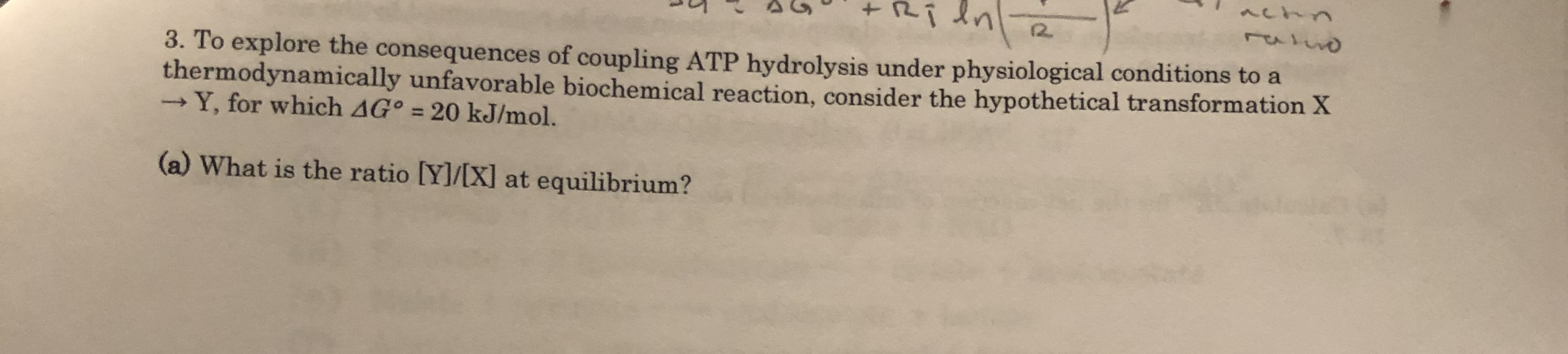# Ri dn12.3. To explore the consequences of coupling ATP hydrolysis under physiological conditions to athermodynamically unfavorable biochemical reaction, consider the hypothetical transformation X-Y, for which AG° = 20 kJ/mol.(a) What is the ratio [Y]/[X] at equilibrium?

Question
14 viewshelp_outlineImage TranscriptioncloseRi dn 12. 3. To explore the consequences of coupling ATP hydrolysis under physiological conditions to a thermodynamically unfavorable biochemical reaction, consider the hypothetical transformation X -Y, for which AG° = 20 kJ/mol. (a) What is the ratio [Y]/[X] at equilibrium? fullscreen
check_circle

Step 1

It is given that the standard Gibb’s free energy for the reaction is 20 kJ/mol.

The reaction is given as follows:

Step 2

Equilibrium constant for a reaction can be expressed in terms of concentration of products to the concentration of reactants raised to some power. It is denoted by K or Kc.

The relation between Gibbs free energy and equilibrium constant can be represented as follows:

Step 3

The equilibrium constant for the given reac...

### Want to see the full answer?

See Solution

#### Want to see this answer and more?

Solutions are written by subject experts who are available 24/7. Questions are typically answered within 1 hour.*

See Solution
*Response times may vary by subject and question.
Tagged in
ScienceChemistry

### Equilibrium Concepts